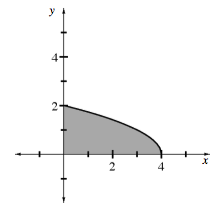### Home > CALC > Chapter 8 > Lesson 8.3.3 > Problem8-126

8-126.
1.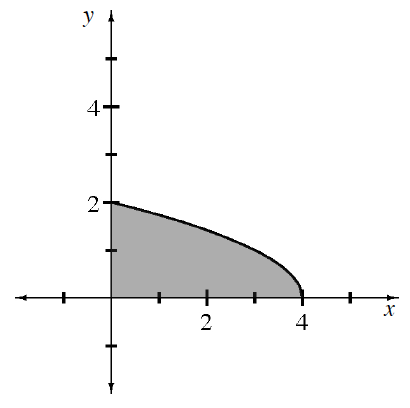Multiple Choice: The base of a solid is a region in the first quadrant under the curve f(x) =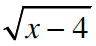. The cross-sections of the solid perpendicular to the x-axis are squares. What is the volume of the solid? Homework Help ✎

1. 8

2.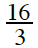3.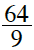4. 8π

5.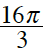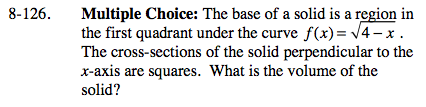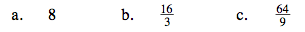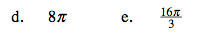$\text{Volume by Cross Sections }=\int_{x=a}^{x=b}(\text{Area function})dx$